# Javaplots

This is a departure from the current set of posts on adding modeling and simulation to Unity game engine. In Unity, I’ve got great built in animation and visualization and have to add my own numerical analysis. In my Intro to Scientific Programming class (first year Java for computational science majors) I have the opposite problem, good tools for handling the math, but not as many options as I’d like for visualization.

There are some good viz tools in Java, but none of them had the ease of use of “plot and pause” in MATLAB, or “plt.plot/plt.show” in python/matplotlib. I needed a simple to use plotting package for basic line plots, scatter plots, histograms, and heatmaps for a first semester group of Java students who were focusing on science applications. I wanted something that could be called in a couple of lines, didn’t require knowledge of exception handling or of swing components or the canvas drawing process in Java, and that could handle either standard arrays or arraylist objects.

I ended up writing a small package, which can be downloaded from https://github.com/joinerda/worldofdata-javaplots.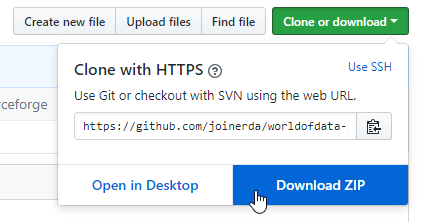Each of the plot objects will create its own JFrame, so students don’t have to learn about graphics or layout to use it–it cant be implemented as easily as a simple dialog box. For students who want to embed the objects inside of an application, this is also possible, and object instantiation differs only by a single boolean variable.

This blog will assume you are creating a java project in eclipse, though the javaplots package will work with or without an IDE. If you are working at a command line, you just need the worldofdata folder in the same files as your java code, or you should be able to add it to any other IDE in whatever way you like to do to manage adding packages to a java project.

To begin, lets start with the hello world of 2D plotting, $y=sin(x)$. I will create a new project in Eclipse with a empty class to create and plot some data, and copy the Javaplots code into the project.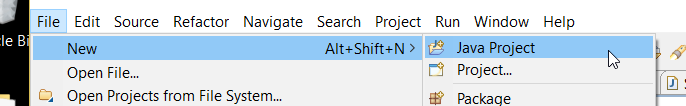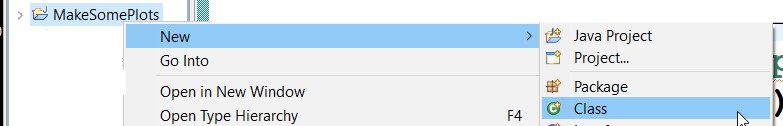The easiest way for a student to link to the Javaplots code is simply to drag the main folder into the source of their project. If you are more comfortable with eclipse and Java in general, you might jar the library up and just drop the jar file in your project, or you might have Javaplots as its own eclipse project and have other java projects include it in their path definitions. As the PERL motto states, TIMTOWTDI! (There is more than one way to do it.)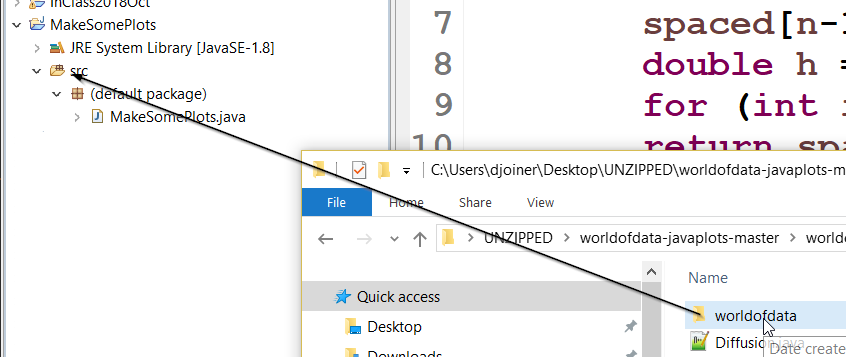In my main method, I will create an array x, linearly spaced from 0 to 2 $\pi$. I’ll write a simple linspace routine to help with this. I’ll also create an array $y$, set equal to $sin(x)$.

public class MakeSomePlots {

public static double [] linspace(double a, double b, int n) {
double [] spaced = new double[n];
spaced = a;
spaced[n-1] = b;
double h = (b-a)/(n-1);
for (int i=1;i<n-1;i++) spaced[i] = a+i*h;
return spaced;
}

public static void main(String[] args) {
int n = 100;
double [] x = linspace(0,2.0*Math.PI,n);
double [] y = new double[n];

for(int i=0;i<n;i++) y[i] = Math.sin(x[i]);

}

}


Now that we have some data, let’s view it. The main plotting objects in Javaplots are SimplePlot (line and marker based scatter plots), SimpleHist (histograms), SimpleBox (box plots), and SimpleGrid (heatmaps).

To create a new plot window, we will instantiate a variable of type SimplePlot using the command “SimplePlot sp = new SimplePlot(500,500);” If you don’t add data to your simple plot window, it will load with default test data. Use the “setData” method to add data, and the setPlotBounds method to set the limits of the plot. Once new information has been added to the plot, it needs to be invalidated so that it will be redrawn, this can be done with the “repaint()” method.

import worldofdata.javaplots.SimplePlot;

public class MakeSomePlots {

public static double [] linspace(double a, double b, int n) {
double [] spaced = new double[n];
spaced = a;
spaced[n-1] = b;
double h = (b-a)/(n-1);
for (int i=1;i<n-1;i++) spaced[i] = a+i*h;
return spaced;
}

public static void main(String[] args) {
int n = 100;
double [] x = linspace(0,2.0*Math.PI,n);
double [] y = new double[n];

for(int i=0;i<n;i++) y[i] = Math.sin(x[i]);

SimplePlot sp = new SimplePlot(500,500);
sp.setData(x, y);
sp.setPlotBounds(0, 2.0*Math.PI, -1, 1);
sp.repaint();

}

}


Run your code, and you should get a plot of $y=sin(x)$.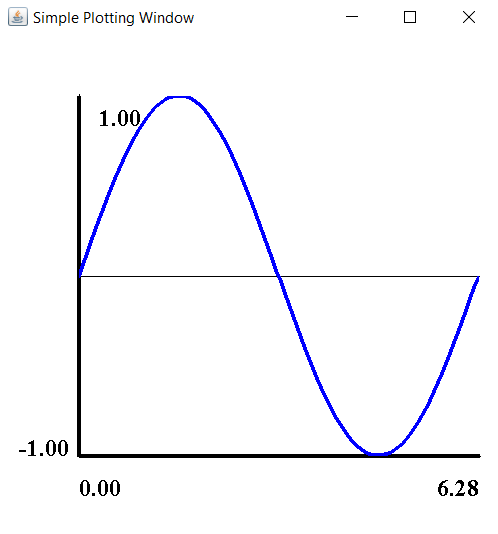You can add additional arguments for line/marker color and plot type (line/marker/both).

sp.setData(x, y,Color.green,SimplePlot.Style.BOTH);


The setData method starts a new plot, but you can add additional data to an existing plot using addData.

To animate a SimplePlot, simple reset the data for the plot and call repaint. You may find that you want to add a delay per frame using the sleep command.

We will make our wave travel, by plotting $sin(x-\omega t)$.

import java.awt.Color;

import worldofdata.javaplots.SimplePlot;

public class MakeSomePlots {

public static double [] linspace(double a, double b, int n) {
double [] spaced = new double[n];
spaced = a;
spaced[n-1] = b;
double h = (b-a)/(n-1);
for (int i=1;i<n-1;i++) spaced[i] = a+i*h;
return spaced;
}

public static void main(String[] args) {
int n = 100;
double [] x = linspace(0,2.0*Math.PI,n);
double [] y = new double[n];
SimplePlot sp = new SimplePlot(500,500);

double t = 0;
double tfinal = 100.0;
double h = .1;
double omega = 2.0;

while(t<tfinal) {
for(int i=0;i<n;i++) y[i] =
Math.sin(x[i]-omega*t);
sp.setData(x, y);
sp.setPlotBounds(0, 2.0*Math.PI, -1, 1);
sp.repaint();
sp.sleep(10);
t += h;
}
}
}


Each of the SimpleGraphComponent applications can also be run as a stand alone application to show a demonstration of the tool, and the main routine for that code can be used as an example of how to implement.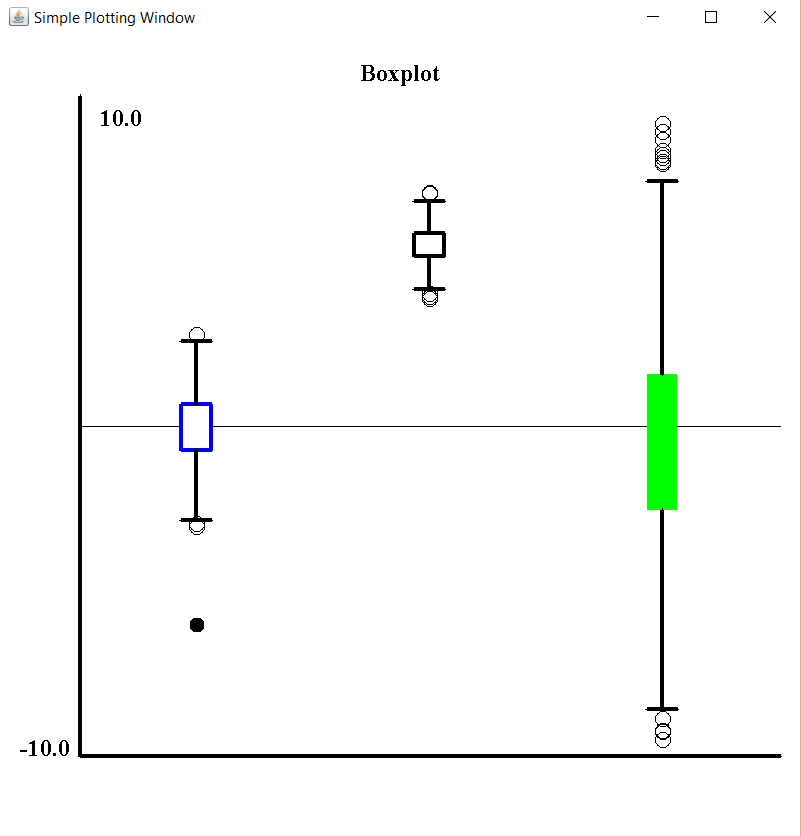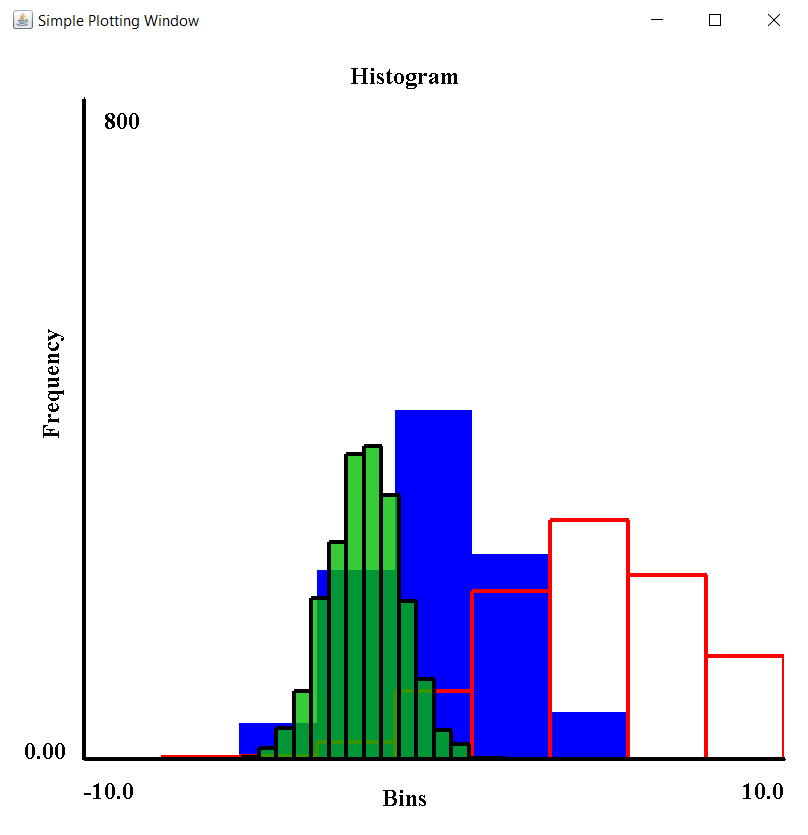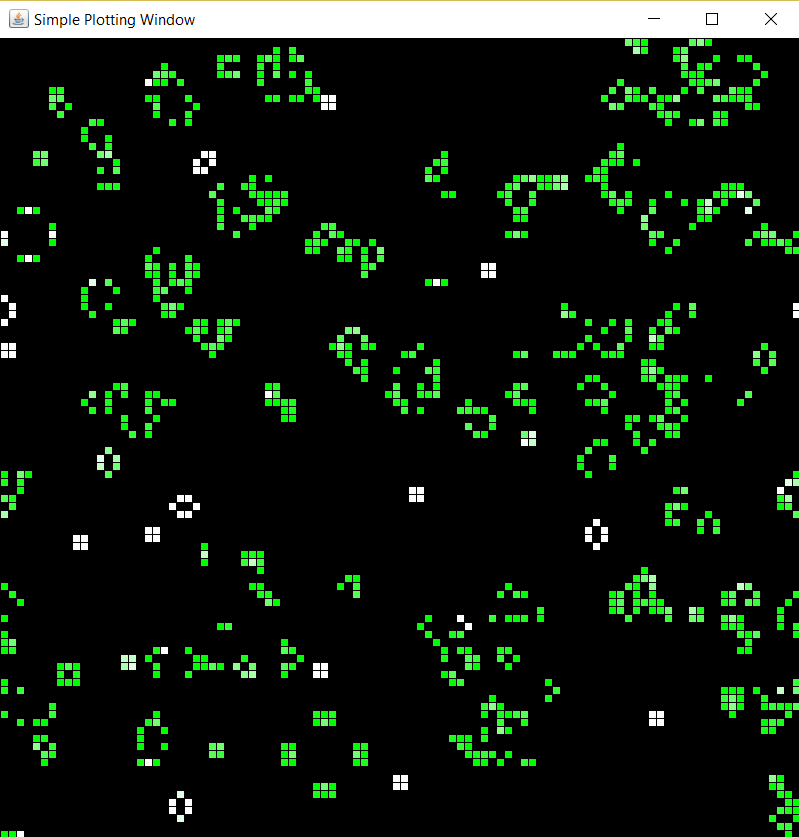There are fancier plotting packages out there, but I found this approach to be useful for me in my introductory Java classes to be able to give my students the ability to plot data we were calculating without having to learn too much about interacting with the graphics system or learning a more complicated professional grade plotting system.

Let me know if you find this helpful or if you use this for any of your classes or plotting needs!